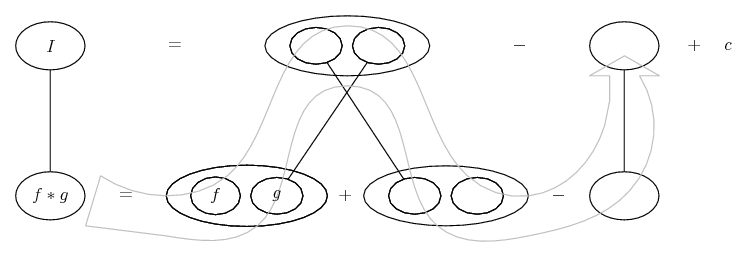# Thread: Calculate the mass M of the truncated cone

1. ##Calculate the mass M of the truncated cone

S={(x ,y ,z) ЄR³ : x² + y² ≤ (2-z)² , 0 ≤ z ≤ 1}

with variable density(x, y, z)= -ln(z)/√x² + y²

I have no clue how to do this...please help...2. ## Re: Calculate the mass M of the truncated cone

Surely you know that the mass of an object is its density integrated over its volume.
Your volume is bounded by the cone $\displaystyle x^2+ y^2= (2- z)^2$, below by z= 0 and above by z= 1. The only thing peculiar about this is that the vertex of the cone is at z= 2. Since z<1 2- z is below 2 and we can use $\displaystyle z= 2- \sqrt{x^2+ y^2}$. Projecting onto the xy-plane, we get circle with center at (0,0) and radii 1 to 2.

In x, y, z coordinates, we would have to integrate over the 'washer' between the circle with radius 1 and the circle with radius 2. That can be done but would require four separate integrals (from x= -2 to x= -1, from x= -1 to 1 with y above the smaller circle, from x= -1 to 1 with y below the smaller circle, from x= 1 to 2). It is much easier to use cylindrical coordinates. In that case, r goes from 1 to 2, $\theta$ goes from 0 to 2\pi, and, for each r and $\theta$, z goes from the plane, z= 1 up to the cone, [tex]z= 2-\sqrt{x^2+ y^2}= 2- \sqrt{r^2}= 2- r.

In cylindrical coordinates the density function is $\displaystyle \frac{-ln(z)}{\sqrt{x^2+ y^2}}= \frac{-ln(z)}{\sqrt{r^2}}= \frac{-ln(z)}{r}$.

That is your integral is $\displaystyle \int_{\theta= 0}^{2\pi}\int_{r= 1}^2\int_{z=1}^{2- r} \frac{-ln(z)}{r}(r drd\theta dz)= -2\pi\int_{r= 1}^{2}\int_{z=1}^{2-r} ln(z)dzdr$.

3. ## Re: Calculate the mass M of the truncated coneOriginally Posted by angel050382S={(x ,y ,z) ЄR³ : x² + y² ≤ (2-z)² , 0 ≤ z ≤ 1}

with variable density(x, y, z)= -ln(z)/√x² + y²
Cylindrical coordinates would simplify things a good deal. The boundary of the cone, in cylindrical form, is

$\displaystyle r^2 = (2-z)^2$

$\displaystyle \Rightarrow r = |2-z| = (2 - z)$ (since $\displaystyle 0\leq z\leq1$ and we want $\displaystyle r\geq0$)

So we have

$\displaystyle 0\leq r\leq 2-z$

$\displaystyle 0\leq\theta\leq2\pi$

and

$\displaystyle 0\leq z\leq 1.$

Now, we integrate the density function over this region to find the mass:

$\displaystyle m = \iiint\limits_S\frac{-\ln z}{\sqrt{x^2+y^2}}\,dV$

$\displaystyle =\int_0^1\int_0^{2\pi}\int_0^{2-z}\frac{-r\ln z}r\,dr\,d\theta\,dz$

$\displaystyle =-\int_0^1\int_0^{2\pi}\int_0^{2-z}\ln z\,dr\,d\theta\,dz$

4. ## Re: Calculate the mass M of the truncated coneOriginally Posted by HallsofIvyThat is your integral is $\displaystyle \int_{\theta= 0}^{2\pi}\int_{r= 1}^2\int_{z=1}^{2- r} \frac{-ln(z)}{r}(r drd\theta dz)= -2\pi\int_{r= 1}^{2}\int_{z=1}^{2-r} ln(z)dzdr$.
Not sure that I agree with your lower bound for $\displaystyle z.$ And if I'm not mistaken, I believe your method would neglect the mass in the center of the frustum (within $\displaystyle r\leq1$).

5. ## Re: Calculate the mass M of the truncated cone

Good point. I think I lost track in the middle and started thinking I was only doing the outside! Thanks for the correction.

6. ## Re: Calculate the mass M of the truncated coneOriginally Posted by HallsofIvyGood point. I think I lost track in the middle and started thinking I was only doing the outside! Thanks for the correction.
I actually very nearly made the same mistake myself, but I caught it before I posted. It's a tricky one.

7. ## Re: Calculate the mass M of the truncated cone

Thank you guys so much!!!! I really appreciate your help. I have another question!

How should I go on now? Cuz if I start to integrate I have problem at ln(0) what is not defined! How should I handle this now!

I know I have a lot of stupid questions, but I really try to pass that math class...:-(

8. ## Re: Calculate the mass M of the truncated cone

Just in case a picture helps work the integral from the inside out...... where (key in spoiler) ...

Spoiler:
Straight lines differentiate downwards with respect to the indicated variable.... is the product rule.... is lazy integration by parts, doing without u and v.

__________________________________________________ __________

Don't integrate - balloontegrate!

Balloon Calculus; standard integrals, derivatives and methods

Balloon Calculus Drawing with LaTeX and Asymptote!

9. ## Re: Calculate the mass M of the truncated coneOriginally Posted by angel050382How should I go on now? Cuz if I start to integrate I have problem at ln(0) what is not defined! How should I handle this now!
Right, I didn't notice that. This will actually be an improper integral:

$\displaystyle =-\int_0^1\int_0^{2\pi}\int_0^{2-z}\ln z\,dr\,d\theta\,dz$

$\displaystyle =-2\pi\int_0^1(2-z)\ln z\,dz$

$\displaystyle =-2\pi\lim_{a\to0^+}\int_a^1(2-z)\ln z\,dz$

$\displaystyle =-2\pi\lim_{a\to0^+}\left[\left(2z-\frac12z^2\right)^2\ln z+\frac14z^2-2z\right]_a^1$ (See tom's post)

$\displaystyle =-2\pi\lim_{a\to0^+}\left[0 + \frac14 - 2 - \left(2a-\frac12a^2\right)\ln a - \frac14a^2 + 2a\right]$

$\displaystyle =-2\pi\lim_{a\to0^+}\left[-\frac74+\left(2a-\frac12a^2\right)\ln a - \frac14a^2 + 2a\right]$

$\displaystyle =-2\pi\left(-\frac74\right) - 2\pi\lim_{a\to0^+}\left(2a-\frac12a^2\right)\ln a$

$\displaystyle =\frac{7\pi}2-\pi\lim_{a\to0^+}\left(4a-a^2\right)\ln a$

$\displaystyle =\frac{7\pi}2-\pi\lim_{a\to0^+}\frac{\ln a}{1/\left(4a-a^2\right)}$

This limit produces the indeterminant form $\displaystyle \textstyle-\frac\infty\infty,$ so we can apply L'Hôpital's rule,

$\displaystyle =\frac{7\pi}2-\pi\lim_{a\to0^+}\frac{1/a}{-(4-2a)/\left(4a-a^2\right)^2}$

$\displaystyle =\frac{7\pi}2+\pi\lim_{a\to0^+}\frac{\left(4a-a^2\right)^2}{a(4-2a)}$

$\displaystyle =\frac{7\pi}2+\pi\lim_{a\to0^+}\frac{a\left(4-a\right)^2}{4-2a}$

$\displaystyle =\frac{7\pi}2.$

calculate, cone, mass, truncated